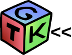gtkIOStream  1.7.0 GTK+ << C++ IOStream operators for GTK+. Now with ORBing, numerical computation, audio client and more ...
findSubSpace.m File Reference

Go to the source code of this file.

size (signal)

## Variables

function eigenvals

signal =signal(:)

ensure a column vector clear s p =round(.25*length(signal))

We need to square the singular values here UHat =U

SHat =S

eigenvectsHat =eigenvects

eigenvalsHat =eigenvals

load tmp results mat stack =dbstack

## ◆ size()

 size ( signal )
Examples:
ALSAExternalPluginTest.C, and BitStreamTest3.C.
Here is the caller graph for this function:

## ◆ eigenvals

 eigenvals
Initial value:
signal
Definition: findSubSpace.m:21

Definition at line 18 of file findSubSpace.m.

## ◆ eigenvalsHat

 eigenvalsHat =eigenvals

Definition at line 30 of file findSubSpace.m.

## ◆ eigenvectsHat

 eigenvectsHat =eigenvects

Definition at line 30 of file findSubSpace.m.

## ◆ p

 ensure a column vector clear s p =round(.25*length(signal))

Definition at line 23 of file findSubSpace.m.

## ◆ SHat

 SHat =S

Definition at line 30 of file findSubSpace.m.

## ◆ signal

 Estimate for a large number of sinusoids this is a correlation matrix signal =signal(:)

Definition at line 21 of file findSubSpace.m.

## ◆ stack

 load tmp results mat stack =dbstack

Definition at line 32 of file findSubSpace.m.

## ◆ UHat

 We need to square the singular values here UHat =U

Definition at line 30 of file findSubSpace.m.

gtkIOStream: /tmp/gtkiostream/mFiles/findSubSpace.m File Reference
 Support this project by purchasing our hardware. GTK+ IOStream  Beta